# Applying Lambda functions to Pandas Dataframe

In Python Pandas, we have the freedom to add different functions whenever needed like lambda function, sort function, etc. We can apply a lambda function to both the columns and rows of the Pandas data frame.

Syntax: lambda arguments: expression

An anonymous function which we can pass in instantly without defining a name or any thing like a full traditional function.

## Applying Lambda Functions to Pandas

Below are some methods and ways by which we can apply lambda functions to Pandas:

• Dataframe.assign() on a Single Column
• Dataframe.assign() on Multiple Columns
• Dataframe.apply() on a Single Row
• Dataframe.apply() on Multiple Rows
• Lambda Function on Multiple Rows and Columns Simultaneously

### Dataframe.assign() on a Single Column

In this example, we will apply the lambda function Dataframe.assign() to a single column. The function is applied to the ‘Total_Marks’ column, and a new column ‘Percentage’ is formed with its help.

## Python3

 `# importing pandas library` `import` `pandas as pd` ` `  `# creating and initializing a list` `values``=` `[[``'Rohan'``,``455``],[``'Elvish'``,``250``],[``'Deepak'``,``495``],` `         ``[``'Soni'``,``400``],[``'Radhika'``,``350``],[``'Vansh'``,``450``]] `   `# creating a pandas dataframe` `df ``=` `pd.DataFrame(values,columns``=``[``'Name'``,``'Total_Marks'``])`   `# Applying lambda function to find ` `# percentage of 'Total_Marks' column ` `# using df.assign()` `df ``=` `df.assign(Percentage ``=` `lambda` `x: (x[``'Total_Marks'``] ``/``500` `*` `100``))`   `# displaying the data frame` `df`

Output: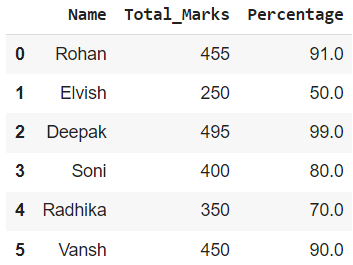Dataframe.assign() on a Single Column

### Dataframe.assign() on Multiple Columns

In this example, we will apply the lambda function Dataframe.assign() to multiple columns. The lambda function is applied to 3 columns i.e., ‘Field_1’, ‘Field_2’, and ‘Field_3’.

## Python3

 `# importing pandas library` `import` `pandas as pd`   `# creating and initializing a nested list` `values_list ``=` `[[``15``, ``2.5``, ``100``], [``20``, ``4.5``, ``50``], [``25``, ``5.2``, ``80``],` `               ``[``45``, ``5.8``, ``48``], [``40``, ``6.3``, ``70``], [``41``, ``6.4``, ``90``],` `               ``[``51``, ``2.3``, ``111``]]`   `# creating a pandas dataframe` `df ``=` `pd.DataFrame(values_list, columns``=``[``'Field_1'``, ``'Field_2'``, ``'Field_3'``])`   `# Applying lambda function to find` `# the product of 3 columns using` `# df.assign()` `df ``=` `df.assign(Product``=``lambda` `x: (x[``'Field_1'``] ``*` `x[``'Field_2'``] ``*` `x[``'Field_3'``]))`   `# printing dataframe` `df`

Output: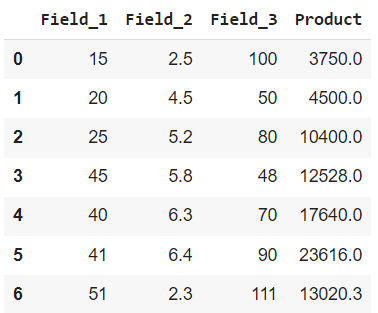Dataframe.assign() on Multiple Columns

### Dataframe.apply() on a Single Row

In this example, we will apply the lambda function Dataframe.apply() to single row. The lambda function is applied to a row starting with ‘d’ and hence square all values corresponding to it.

## Python3

 `# importing pandas and numpy libraries` `import` `pandas as pd` `import` `numpy as np`   `# creating and initializing a nested list` `values_list ``=` `[[``15``, ``2.5``, ``100``], [``20``, ``4.5``, ``50``], [``25``, ``5.2``, ``80``],` `               ``[``45``, ``5.8``, ``48``], [``40``, ``6.3``, ``70``], [``41``, ``6.4``, ``90``], ` `               ``[``51``, ``2.3``, ``111``]]`   `# creating a pandas dataframe` `df ``=` `pd.DataFrame(values_list, columns``=``[``'Field_1'``, ``'Field_2'``, ``'Field_3'``],` `                  ``index``=``[``'a'``, ``'b'``, ``'c'``, ``'d'``, ``'e'``, ``'f'``, ``'g'``])`     `# Apply function numpy.square() to square` `# the values of one row only i.e. row ` `# with index name 'd'` `df ``=` `df.``apply``(``lambda` `x: np.square(x) ``if` `x.name ``=``=` `'d'` `else` `x, axis``=``1``)`     `# printing dataframe` `df`

Output: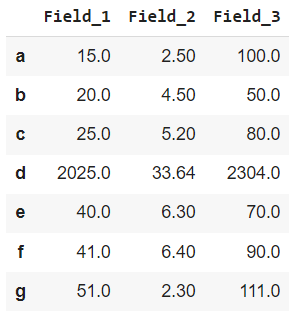Dataframe.apply() on a Single Row

### Dataframe.apply() on Multiple Rows

In this example, we will apply the lambda function to multiple rows using Dataframe.apply(). The lambda function is applied to 3 rows starting with ‘a’, ‘e’, and ‘g’.

## Python3

 `# importing pandas and numpylibraries` `import` `pandas as pd` `import` `numpy as np`   `# creating and initializing a nested list` `values_list ``=` `[[``15``, ``2.5``, ``100``], [``20``, ``4.5``, ``50``], [``25``, ``5.2``, ``80``],` `               ``[``45``, ``5.8``, ``48``], [``40``, ``6.3``, ``70``], [``41``, ``6.4``, ``90``],` `               ``[``51``, ``2.3``, ``111``]]`   `# creating a pandas dataframe` `df ``=` `pd.DataFrame(values_list, columns``=``[``'Field_1'``, ``'Field_2'``, ``'Field_3'``],` `                  ``index``=``[``'a'``, ``'b'``, ``'c'``, ``'d'``, ``'e'``, ``'f'``, ``'g'``])`     `# Apply function numpy.square() to square ` `# the values of 3 rows only i.e. with row` `# index name 'a', 'e' and 'g' only` `df ``=` `df.``apply``(``lambda` `x: np.square(x) ``if` `x.name ``in` `[` `              ``'a'``, ``'e'``, ``'g'``] ``else` `x, axis``=``1``)`   `# printing dataframe` `df`

Output: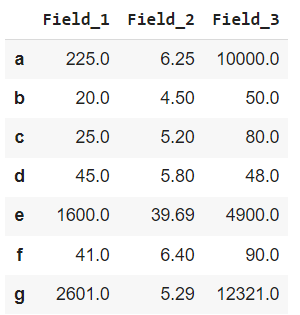Dataframe.apply() on Multiple Rows

### Lambda Function on Multiple Rows and Columns Simultaneously

In this example, we will apply the lambda function simultaneously to multiple columns and rows using dataframe.assign() and dataframe.apply().

## Python3

 `# importing pandas and numpylibraries` `import` `pandas as pd` `import` `numpy as np`   `# creating and initializing a nested list` `values_list ``=` `[[``1.5``, ``2.5``, ``10.0``], [``2.0``, ``4.5``, ``5.0``], [``2.5``, ``5.2``, ``8.0``],` `               ``[``4.5``, ``5.8``, ``4.8``], [``4.0``, ``6.3``, ``70``], [``4.1``, ``6.4``, ``9.0``],` `               ``[``5.1``, ``2.3``, ``11.1``]]`   `# creating a pandas dataframe` `df ``=` `pd.DataFrame(values_list, columns``=``[``'Field_1'``, ``'Field_2'``, ``'Field_3'``],` `                  ``index``=``[``'a'``, ``'b'``, ``'c'``, ``'d'``, ``'e'``, ``'f'``, ``'g'``])`     `# Apply function numpy.square() to square ` `# the values of 2 rows only i.e. with row` `# index name 'b' and 'f' only` `df ``=` `df.``apply``(``lambda` `x: np.square(x) ``if` `x.name ``in` `[``'b'``, ``'f'``] ``else` `x, axis``=``1``)`   `# Applying lambda function to find product of 3 columns` `# i.e 'Field_1', 'Field_2' and 'Field_3'` `df ``=` `df.assign(Product``=``lambda` `x: (x[``'Field_1'``] ``*` `x[``'Field_2'``] ``*` `x[``'Field_3'``]))`     `# printing dataframe` `df`

Output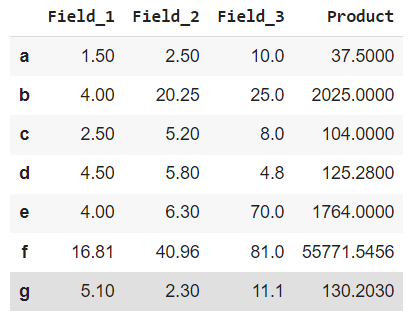Lambda Function on Multiple Rows and Columns Simultaneously

Whether you're preparing for your first job interview or aiming to upskill in this ever-evolving tech landscape, GeeksforGeeks Courses are your key to success. We provide top-quality content at affordable prices, all geared towards accelerating your growth in a time-bound manner. Join the millions we've already empowered, and we're here to do the same for you. Don't miss out - check it out now!

Previous
Next